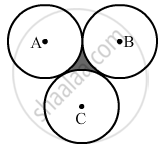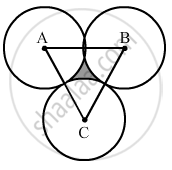Advertisement Remove all ads

# Three Equal Circles, Each of Radius 6 Cm, Touch One Another as Shown in the Figure. Find the Area of Enclosed Between Them. - Mathematics

Sum

Three equal circles, each of radius 6 cm, touch one another as shown in the figure. Find the area of enclosed between them.Advertisement Remove all ads

#### SolutionJoin ABC. All sides are equal, so it is an equilateral triangle.
Now,
Area of the equilateral triangle =sqrt(3)/4xx"Side"^2

= 1.73/4xx12xx12

= 62.28 cm

Area of the arc of the circle=60/360pi"r"^2

= 1/6pi"r"^2

=1/6xx22/7xx6xx6

= 18.86 cm2

Area of the three sectors = 3 × 18.86 = 56.57 cm

Area of the shaded portion = Area of the triangle -- Area of the three quadrants

= 62.28 - 56.57

= 5.71 cm

Is there an error in this question or solution?
Advertisement Remove all ads

#### APPEARS IN

RS Aggarwal Secondary School Class 10 Maths
Chapter 18 Area of Circle, Sector and Segment
Exercise 18B | Q 39 | Page 833
Advertisement Remove all ads

#### Video TutorialsVIEW ALL 

Advertisement Remove all ads
Share
Notifications

View all notifications

Forgot password?